View Part: 1 2 3 4 5 6 7 8 9 10 11 12

PART 7 - LINES THROUGH THE EARTH

The alignment of ancient sites may be viewed as a circle because all of the sites are on a straight line around the center of the earth. The two points where the circle crosses the equator are on the horizontal axis, and the two points where the circle reaches the highest latitudes are on the vertical axis. The center of the circle is the center of the earth.

The distance from the center of the earth to any point on the circle is the radius of the earth (24,892 ÷ π ÷ 2 = 3962 miles).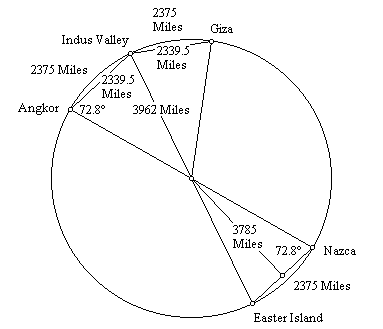The great circle distance from Easter Island to Nazca is 2375 miles, or 9.54% of the great circle (2375/24,892 = 09.54) or 34.344° (.0954 x 360 = 34.344°). This also gives 34.344° for the angle between the two sites at the center of the earth. One half of this angle is 17.172°.The straight line distance between the two sites is equal to the sine of one half of their angle at the center of the earth times the diameter of the earth. The sine of 17.172° is .29524.

The diameter of the earth (7924 miles) times .29524 gives 2339.5 miles for the straight line (through the earth) distance from Easter to Nazca. The height of a triangle with sides of 3962 miles and a baselength of 2339.5 miles is 3785 miles. The baselength of the triangle times φ is equal to the height of the triangle (2339.5 x 1.618 = 3785). The center of the earth, Angkor and Easter’s antipodal point in the Indus Valley forms this same φ triangle, as does the triangle formed by the center of the earth, the Indus Valley and Giza.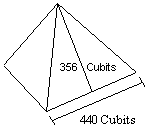The length of the base of each face of the Great Pyramid is 440 cubits. The slant height of each face is 356 cubits. One half of the length of the base times φ equals the slant height of the Great Pyramid:

440 cubits ÷ 2 = 220 cubits

220 cubits x 1.618 = 356 cubits

The ratio of the base to the slant height of the Great Pyramid is exactly two times the ratio of the base to the height of the throught the earth triangles shown above.

Just south of Giza, the step pyramid at Saqqara is believed to have been the first stone pyramid built in Egypt.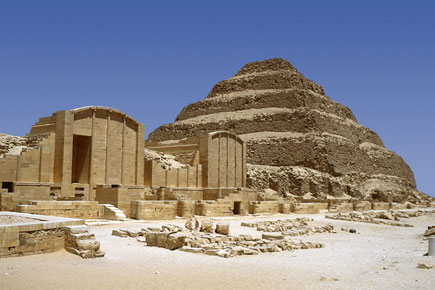The steps of this pyramid have been estimated to be inclined to the vertical in the range of 16°-18°. Recent measurements by Jon Bodsworth indicate a 17° angle of inclination. Measurements by Robert Bauval also indicate an angle of 17° with a margin of error of plus or minus 20'.

An inclination of 17.172° (17° 10') to the vertical gives an inclination of 72.828° to the horizontal, the same as the through earth terrestrial triangles shown above: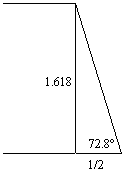The 2φ ratio between the base and the height of the right triangle formed by the inclination of the pyramid steps is the same as the ratio between the half-base and the height of the through earth terrestrial triangles shown above.

10 miles southwest of Easter Island is a point along the great circle alignment that is equally distant at 10,071 miles from Giza and Angkor Vihear. Through earth lines from this point to Angkor and Giza are 7571 miles long. A great circle distance from Giza to Angkor of 4750 miles converts to a straight line distance of 4470.5 miles. The height of this straight line triangle is 7233.5 miles.

The base angles of the triangle at Giza and Angkor are 72.828° and the φ ratio between the height and the base of the triangle is the same as the through earth triangles shown above: 4470.5 x 1.618 = 7233.5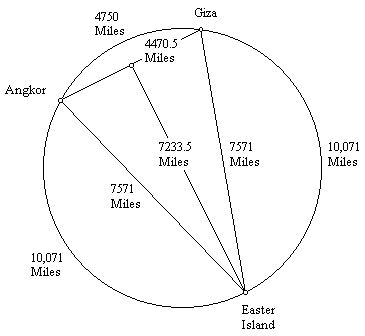The straight line distance from Easter Island to Machupicchu is 2522 miles (great circle distance: 2566 miles). The straight line distance from Easter to it's antipodal point in the Indus Valley is 7924 miles (diameter of the great circle). The straight line distance from Easter to Giza is 7566 miles and the straight line distance from Easter to Angkor Wat is 7574 miles.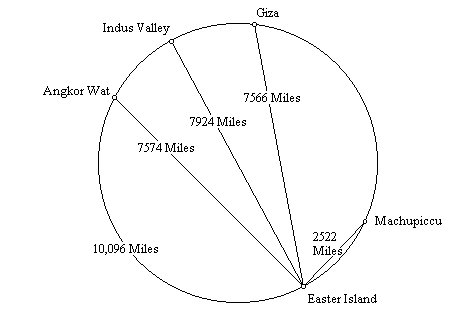The straight line distance, through the Earth, from Angkor Wat to Easter (7,574 miles), plus the straight line distance from Easter to Macchupicchu (2,522 miles), equals the great circle distance from Angkor Wat to Easter (10,096 miles).

The straight line distance from the Great Pyramid to Easter (7,566 miles) is three times the straight line distance from Easter to Machupicchu (2,522 miles).

The straight line distance from Easter to its antipodal point in the Indus Valley (7,924 miles), which is also the diameter of the Earth, is 3.1416 times the straight line distance from Easter to Machupicchu (2,522 miles), a precise expression of π.

Since the circumference of the Earth is also 3.1416 times the diameter of the Earth, the straight line distance from Easter to Machupicchu times π˛ equals the circumference of the Earth.